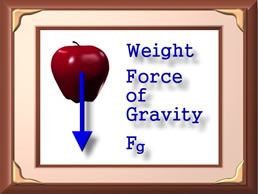# Difference Between Density and Weight

##### Key difference: Density is the object’s mass per volume. Weight is the force that the earth pulls on the mass of the object.Density and weight are both terms most commonly used in physics, however for the general public, the difference between the two may not be familiar. This is made even more difficult as the terms also have other uses in other fields. Such as weight is also used in the health and medical arena for personal weight, or as density is also used to refer to population density in statistics.

Density can be defined as “the physical property of matter that deals with the mass of an object per volume in itself.” Mass is the measure of the amount of matter in an object, volume is the object’s height, width, and length. Basically, density is mass per volume. It is a physical property of matter, which measures how much matter is there in a given volume of an object. The measuring unit for density may be kilograms per cubic meter (kg/m-3) or grams per millilitre (g/ml).

The metric system was designed so that water will have a density of one gram per cubic centimeter. Every other object is compared to this scale. For example, lead is about 10 times as dense as water and Styrofoam is about one tenth as dense as water. If lead is placed in water, it will sink to the bottom, whereas, if Styrofoam is placed in water, it will float on top of it.

Density is considered as an intensive property, that is, it doesn’t change with the size or location of an object. However, density is subject to environmental changes like pressure and temperature. If the temperature of the object or the atmospheric pressure applicable on the object changes, it will have an impact on its density. This is the reason that hot air tends to rise above normal air, a concept that hot air balloons, operate on.On the other hand, the weight of a mass is the force of gravity on the mass. It can also be said that the weight of a mass is the force that the earth pulls on the mass. Like density, weight is also considered to be a physical property of matter. It concerns the mass of an object with consideration to the force that affects that object. Unlike density, weight of an object is an extensive property. It changes with the object’s size or location. For example, our weight is the force that the earth pulls on us. So, if we get further away from the earth, the force with which the earth pulls on us gets less effective and hence we weigh less. Weight is measured in Newtons or kilogram weight (kgwt).

For an easier way to differentiate between weigh and density, note this example: A ton of iron and a ton of straw weigh the same, that is a ton, but the two objects tend to take up different amounts of space because they have different densities.